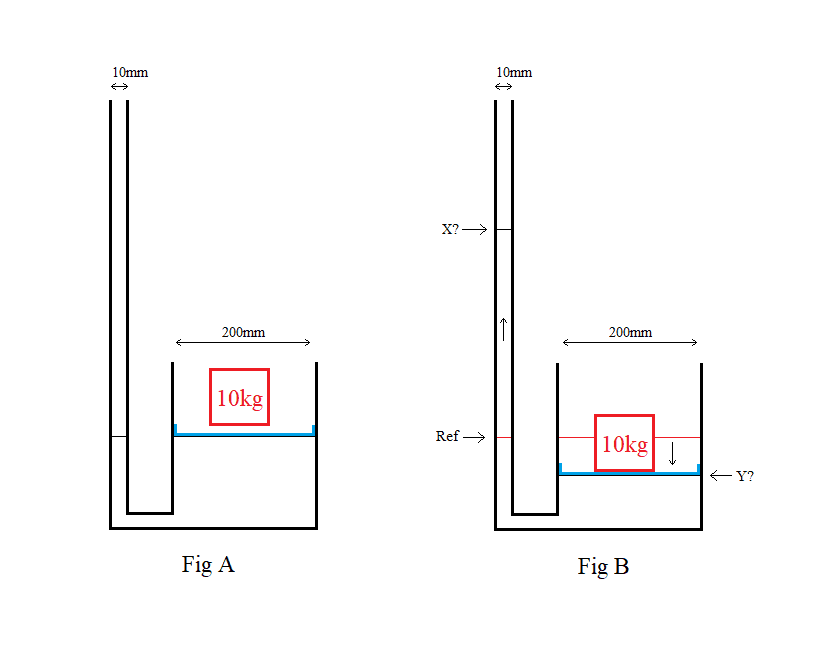# Fluid pressure

NO TEMPLATE BECAUSE ORIGIONALLY SUBMIIED TO NON-homework forum

Hi,

I have a pipe connects to a container's outlet. The container contains water and the pipe is open-ended. When a 10Kg object is placed inside the container and compress the water. Can you tell me how to calculate the displacement of X and Y? What principle or formula should I use?Thanks,

Last edited by a moderator:

Chestermiller
Mentor
What is your assessment so far?

What is your assessment so far?
Someone told me the "extra" water weight inside the pipe will be equal to the weight of the 10kg object. (it seems make sense)

10kg = 0.01 cubic meters of water.
Using Vol to cal the cylinder height:
X = 127.48m
Y = 0.32m

Is that correct?

Chestermiller
Mentor
Someone told me the "extra" water weight inside the pipe will be equal to the weight of the 10kg object. (it seems make sense)

10kg = 0.01 cubic meters of water.
Using Vol to cal the cylinder height:
X = 127.48m
Y = 0.32m

Is that correct?
No. This is not correct.

In Fig. B, what is the pressure that the piston (located beneath the 10 kg mass) exerts on the fluid immediately below it (neglecting the weight of the piston)?

Last edited:
No. This is not correct.

In Fig. B, what is the pressure that the 10 kg mass exerts on the piston?
F = ma = 10kg * 9.8 = 98N
P = F/A = 98/(3.14 * 0.1 * 0.1) = 3121 Pascals

Chestermiller
Mentor
F = ma = 10kg * 9.8 = 98N
P = F/A = 98/(3.14 * 0.1 * 0.1) = 3121 Pascals
Excellent. Now, in terms of X and Y, what is the height of the water in the left column (of B) above the level of the piston? Algebraically, in term so of X and Y, what is the fluid pressure in this column at the same level as the piston?

Excellent. Now, in terms of X and Y, what is the height of the water in the left column (of B) above the level of the piston? Algebraically, in term so of X and Y, what is the fluid pressure in this column at the same level as the piston?
P = ρgh
h = P/ρg
= 3121 Pa / (997 kg/m³ * 9.8)
= 0.32m

So the pressure from the 10Kg can only push the X, 0.32m upward instead of 127.48m. Is that correct?

Chestermiller
Mentor
P = ρgh
h = P/ρg
= 3121 Pa / (997 kg/m³ * 9.8)
= 0.32m

So the pressure from the 10Kg can only push the X, 0.32m upward instead of 127.48m. Is that correct?
Not quite. That is (X + Y)=0.32 m
Since the volume displaced upward in the left column must equal to the volume displaced downward in the right column, how are X and Y related?